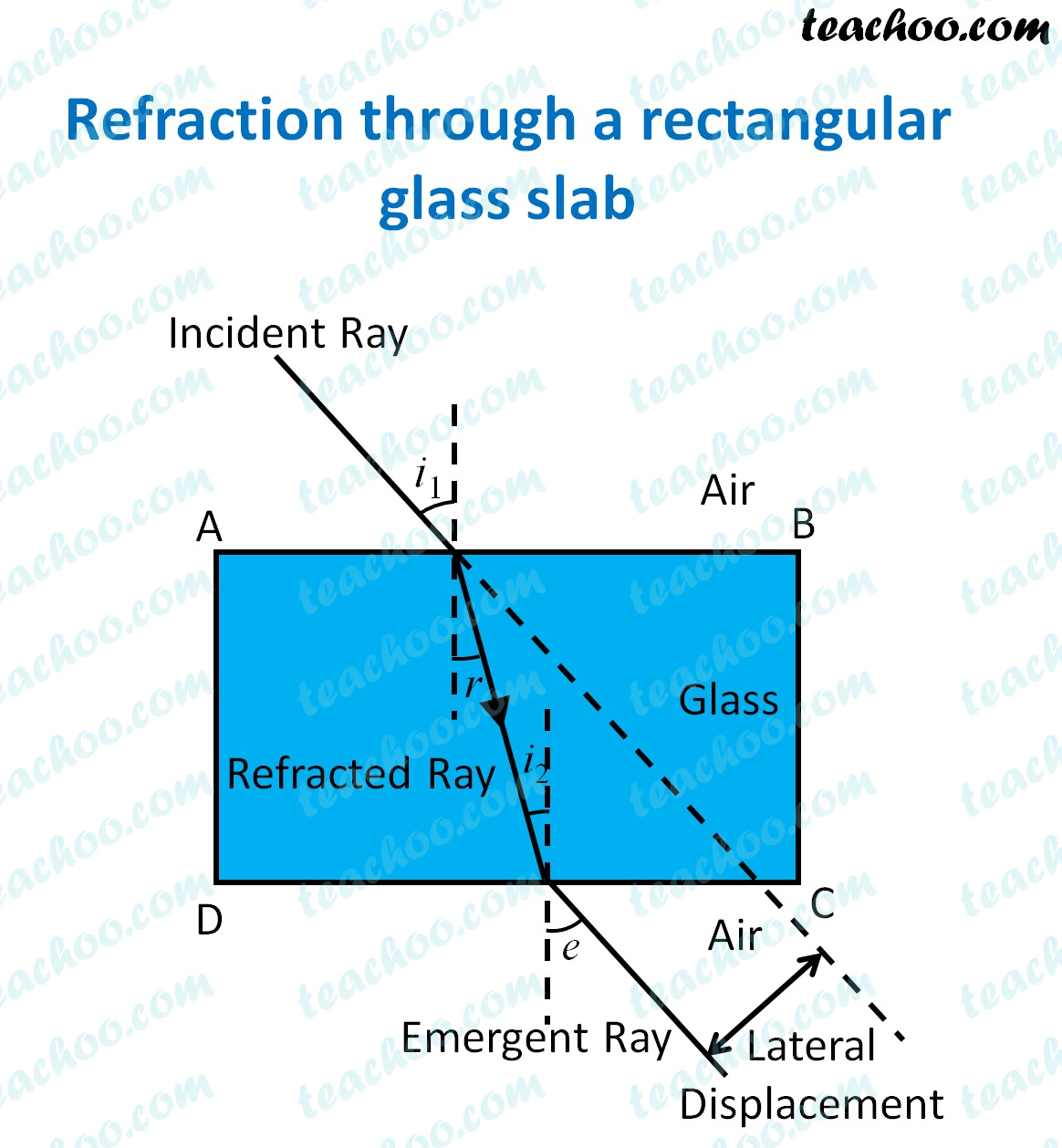MCQ from Past Year Papers

Class 10
Chapter 10 Class 10 - Light - Reflection and Refraction (Term 1)

## (d) ∠i = ∠e < ∠r• For a glass slab, light enters from rarer to denser medium, hence ray is bent towards from normal
• Thus, angle of refraction will be less than angle of incidence
• Then, when it comes out, light travels from denser to rarer medium, hence ray bends away from normal
• Hence, angle of emergence is greater than angle of refraction.
• Since opposite faces of glass slab are parallel, angle of emergence will be equal to angle of incidence

So, the correct answer is (b) - ∠i = ∠e > ∠r